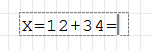# Expression

Write an Expression in RedCrab Calculator

## Basics

• Mathematical expressions can be written on any position on the work sheet.

• Any number of expressions can be written to the worksheet.

• A calculation starts at the top of the worksheet without a given order. RedCrab first computes the expressions whose results are used in other expressions. This logical order allows you to use variables at the top of the worksheet that are defined or calculated below.

• A result is displayed only for expressions that complete with an equal sign.## Frames

• If you write a expression, RedCrab draws a frame fit to the formula size.

• Each formula has its own frame. Use the frame to move the formulas with the mouse in the worksheet.## Input hints

• The expression frame can be moved with the mouse.

• Shift + Insert inserts a new row. This moves all frames below the cursor position down one row.

• Shift + Delete deletes a row. To do this, all of the following frames are shifted up one row.

• Press Home to move the cursor to the first column of the frame or to the first column of the worksheet.

• Press End key to move the cursor to the last column of the frame or to the next frame of the row.

• Press Shift + Enter to move the cursor to the first column below the frame.